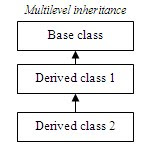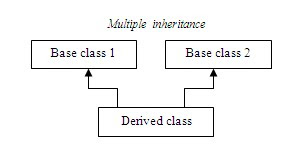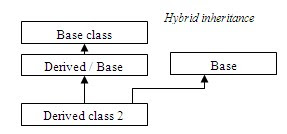/* related posts with thumb nails */

###Forms (Types) of Inheritance:

4 Inheritance is the process of deriving a new class from an existing class.

4 Inheritance can be formed mainly in five ways:

§ Single inheritance

§ Multilevel inheritance

§ Multiple inheritance

§ Hierarchical inheritance

§ Hybrid Inheritance

Single inheritance:

If a class is derived from another class, it can be called as single inheritance.Syntax:

class A

{

}

class B extends A

{

}

Example:

class alpha

{

public void show1()

{

System.out.println(“hello alpha”);

}

}

class beta extends alpha

{

public void show2()

{

System.out.println(“hello beta”);

}

}

class Test

{

public static void main(String as[])

{

beta b1=new beta();

b1.show1(); // prints “hello alpha”

b1.show2(); // prints “hello beta”

}

}

Multilevel inheritance:

If a class is derived from the class, which is derived from another class, it can be called as multilevel inheritance.Syntax:

class A

{

}

class B extends A

{

}

class C extends B

{

}

Example:

class alpha

{

public void show1()

{

System.out.println(“hello alpha”);

}

}

class beta extends alpha

{

public void show2()

{

System.out.println(“hello beta”);

}

}

class gamma extends beta

{

public void show3()

{

System.out.println(“hello gamma”);

}

}

class Test

{

public static void main(String as[])

{

gamma g1=new gamma();

g1.show1(); // prints “hello alpha”

g1.show2(); // prints “hello beta”

g1.show3(); // prints “hello gamma”

}

}

Multiple inheritance:

If a class is derived from two or more classes, it can be called as multiple inheritance. Java does not support this type of inheritance.Hierarchical inheritance:

 Hierarchical inheritance
 Derived class 2
If two or more classes are derived from a class, it can be called as hierarchical inheritance.

Syntax:

class A

{

}

class B extends A

{

}

class C extends A

{

}

Example:

class alpha

{

public void show1()

{

System.out.println(“hello alpha”);

}

}

class beta extends alpha

{

public void show2()

{

System.out.println(“hello beta”);

}

}

class gamma extends beta

{

public void show3()

{

System.out.println(“hello gamma”);

}

}

class Test

{

public static void main(String as[])

{

beta b1 = new beta();

b1.show1(); // prints “hello alpha”

b1.show2(); // prints “hello beta”

gamma g1= new gamma();

g1.show1(); // prints “hello alpha”

g1.show3(); // prints “hello gamma”

}

}

Hybrid inheritance:

This could be the combination of multiple and multilevel inheritance. Java does not support this type of inheritance.Base class 1
 Multiple inheritance
 Base class 2

Related Topics: